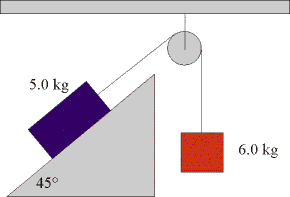# Newton's Laws of motion

Two blocks (5.0 kg and 6.0 kg) are connected through a frictionless system. Find the tension in the string and the acceleration of the system. The angle of the ramp is 45degrees.I have taken the direction of motion of each mass as the positive side.
So this is what I did,
For m=6kg
Fnety= Fg-T=6a
Fg-T=6a

For m= 5 Kg
Fnetx= T-Ff=5a
This is what i could do. I am unclear on how to use the angle..Any help?

Doc Al
Mentor
For m= 5 Kg
Fnetx= T-Ff=5a
What is Ff? (There's no friction.) Don't forget the component of gravity parallel to the incline. (That's where the angle comes in.)

uhh..so it will be something like this?
For m=5Kg
Fa=mgSinTheta and Fn=mgcosTheta?

Doc Al
Mentor
Yes, the component of the weight parallel to the ramp is $mg\sin\theta$ and the component perpendicular to the ramp is $mg\cos\theta$. Since the acceleration is parallel to the ramp, that's the component that you want.

so, mgsinTheta is the only force for m=5kg, we have to take into account?

Doc Al
Mentor
so, mgsinTheta is the only force for m=5kg, we have to take into account?
No, you still have the rope pulling it up the ramp.

yea so the net force is T-mgsinTheta?

Doc Al
Mentor
yea so the net force is T-mgsinTheta?
Yes.

one more question: a similar type
Three blocks (12 kg, 6.0 kg and 4.0 kg) are connected through a frictionless system. Find the tension in the two strings and the acceleration of the system.

So for the acceleration:
Fnetx of system=T=ma
T1+T2=6a
T1=6a-T2

Fy= 117.6-T1 (for m=12kg)
Fy= T2-39.2 (for m=4 kg)
Net Fy for the system= 78.4+T2-T1=ma
78.4+T2-6a+T2=16a
78.4+2T2= 22a
This is where i got stuck

#### Attachments

Doc Al
Mentor
Attack it systematically. Call the acceleration "a" and assume the system moves "to the left". We'll take that direction to be positive. Now analyze the forces on each mass and apply Newton's 2nd law.
So for the acceleration:
Fnetx of system=T=ma
T1+T2=6a
T1=6a-T2
The net force on the 6 kg mass is: T1 - T2 (note the minus sign, since the tensions pull in opposite directions)
So Newton II tells us:
T1 - T2 = 6a [equation 1]

Fy= 117.6-T1 (for m=12kg)
That's good. Now apply Newton II:
117.6-T1 = 12a [equation 2]
Fy= T2-39.2 (for m=4 kg)
Also good. Newton II:
T2-39.2 = 4a [equation 3]

Now combine those three equations and solve for the three unknowns. (Hint: Just add the equations together and see what happens.)